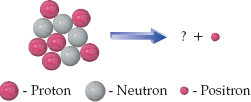×
Get Full Access to Introductory Chemistry - 5 Edition - Chapter 17 - Problem 101p
Get Full Access to Introductory Chemistry - 5 Edition - Chapter 17 - Problem 101p

×

# Solved: Closely examine the diagram representing the positron emission of carbon-10 andISBN: 9780321910295 34

## Solution for problem 101P Chapter 17

Introductory Chemistry | 5th Edition

• Textbook Solutions
• 2901 Step-by-step solutions solved by professors and subject experts
• Get 24/7 help from StudySoup virtual teaching assistantsIntroductory Chemistry | 5th Edition

4 5 1 279 Reviews
20
2
Problem 101P

Closely examine the diagram representing the positron emission of carbon-10 and draw the missing nucleus.Step-by-Step Solution:
Step 1 of 3

Solution 101P:

Here, we are going to draw the missing nucleus.

Step1:

In a nuclear reaction, the sum of the superscripts (mass numbers, A) for the reactants must be equal to the sum of the superscripts for the products. Similarly, the sum of the subscripts (atomic numbers, Z) for the reactants must be equal to the sum of the subscripts for the products.

Step2:

The nucleus given in the reactant side has 6 protons, i.e., its atomic number is 6. Thus the nucleus isC.

The positron is represented by the symbole.

Thus,...

Step 2 of 3

Step 3 of 3

##### ISBN: 9780321910295

Introductory Chemistry was written by and is associated to the ISBN: 9780321910295. The answer to “Closely examine the diagram representing the positron emission of carbon-10 and draw the missing nucleus.” is broken down into a number of easy to follow steps, and 15 words. Since the solution to 101P from 17 chapter was answered, more than 632 students have viewed the full step-by-step answer. The full step-by-step solution to problem: 101P from chapter: 17 was answered by , our top Chemistry solution expert on 05/06/17, 06:45PM. This full solution covers the following key subjects: carbon, closely, diagram, draw, emission. This expansive textbook survival guide covers 19 chapters, and 2045 solutions. This textbook survival guide was created for the textbook: Introductory Chemistry, edition: 5.

Unlock Textbook Solution